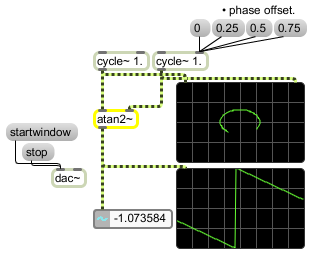# atan2~

Signal arc-tangent function (two variables)

## Description

acosh~ will take two given x and y values and output a signal which is their arc-tangent function, calculated as follows:
Arc-tangent (y/x)

None.

## Messages

 int y and x-values [int] Instead of a signal, atan2~ can also take integers in either inlet to specify the x and y values in the same way a signal would but without sample-accurate timing. float y and x-values [float] Instead of a signal, atan2~ can also take floats in either inlet to specify the x and y values in the same way a signal would but without sample-accurate timing. signal In left input: y value input to an arc-tangent function. In right input: x value input to an arc-tangent function.

## Output

signal: The arc-tangent input values (i.e. Arc-tangent (y/x)).

## ExamplesCalculate the angle of two points around an origin (0 0) in radians